• 三维向量叉乘的推导老是记不住，这里看了一篇很好的文章，记录一下： https://www.cnblogs.com/qilinzi/archive/2013/05/09/3068158.html
三维向量叉乘的推导老是记不住，这里看了一篇很好的文章，记录一下：
https://www.cnblogs.com/qilinzi/archive/2013/05/09/3068158.html
展开全文机器学习
• 一直以来，我都记不住向量叉乘的结果，每次都要查询。其根本原因在于，我没有去研究过叉乘是如何推导出来的。于是，这次想彻底解决一下。首先要感谢维基百科，它已经把所有问题都描述清楚了。 ... ...


一直以来，我都记不住向量叉乘的结果，每次都要查询。其根本原因在于，我没有去研究过叉乘是如何推导出来的。于是，这次想彻底解决一下。首先要感谢维基百科，它已经把所有问题都描述清楚了。
http://en.wikipedia.org/wiki/Cross_product

而下面的文字，只是我的读书笔记，以加深自己的印象。

首先我们知道 ，对于向量u和v, u x v的结果，是得到一个既垂直于u又垂直于v的向量,假设记作n.
则有下面公式
n = u x v;
而n的方向，是由右手法则决定的。 即伸出右手，四个手指方向从u绕到v. 此时，大姆指的方向，就是n的方向。 我们通常叫做右向量。
引用一下维基百科的图来说明问题，有兴趣的兄弟可以照图比划一下。 （注：图中向量是用的a x b来表示）有了上面的知识，我们继续向下看。
我们假设向量 u,v,n分别用三个标量来表示。即
u = (Xu,Yu,Zu)
v = (Xv,Yv,Zv)
n = (Xn,Yn,Zn)
则，它们的关系为
Xn = Yu*Zv – Zu*Yv;
Yn = Zu*Xv – Xu*Zv;
Zn = Xu*Yv – Yu*Xv;
即 n = (Yu*Zv – Zu*Yv,Zu*Xv – Xu*Zv,Xu*Yv – Yu*Xv);
而为了验证n与u和v的垂直性，可以使用点乘进行
点乘法则比这个简单多了， u*v = (Xu*Xv + Yu*Yv + Zu*Zv) = dotUV;
如果两个向量垂直，则dotUV = 0;
代入验证一把
u*n = (Xu*(Yu*Zv – Zu*Yv) + Yu*(Zu*Xv – Xu*Zv) + Zu*(Xu*Yv – Yu*Xv));
= Xu*Yu*Zv – Xu*Zu*Yv + Yu*Zu*Xv – Yu*Xu*Zv + Zu*Xu*Yv – Zu*Yu*Xv;
把正负号的因式仔细比对一下，发现刚好可以低消。 结果为0.
v*n 同理可证。
于是，也验证了n与u,v垂直的特性。

如果只是为了应用的话，走到这一步就可以停下了。后面的知识，只是为了满足一下好奇心。
那我们就来看看，这个结论是怎么来的呢？ 我们接着来推导。
为了更好地推导，我们需要加入三个轴对齐的单位向量。
i,j,k.
i,j,k满足以下特点
i = j x k; j = k x i; k = i x j;
k x j = –i; i x k = –j; j x i = –k;
i x i = j x j = k x k = 0; (0是指0向量）
由此可知，i,j,k是三个相互垂直的向量。它们刚好可以构成一个坐标系。
这三个向量的特例就是 i = (1,0,0) j = (0,1,0) k = (0,0,1)。

好，那对于处于i,j,k构成的坐标系中的向量u,v我们可以如下表示
u = Xu*i + Yu*j + Zu*k;
v = Xv*i + Yv*j + Zv*k;

那么 u x v = (Xu*i + Yu*j + Zu*k) x (Xv*i + Yv*j + Zv*k)
= Xu*Xv*(ixi) + Xu*Yv*(i x j) + Xu*Zv*(i x k) + Yu*Xv*(j x i) + Yu*Yv*(j x j) + Yu*Zv*(j x k) + Zu*Xv*( k x i ) + Zu*Yv(k x j) + Zu*Zv(k x k)
由于上面的i,j,k三个向量的特点，所以，最后的结果可以简化为
u x v = (Yu*Zv – Zu*Yv)*i + (Zu*Xv – Xu*Zv)j + (Xu*Yv – Yu*Xv)k;
于是，在i,j,k构成的坐标系中。集就是上面的结果。
当i = (1,0,0) j = (0,1,0) k = (0,0,1)时，我们通常省略i,j,k的写法。最终也就得到了我们的右向量。

叉乘的意义
叉乘表示垂直于uxv的右向量。
使用的地方
可以通过叉乘，修正向量关系，从而构建坐标系。 常见的有 摄相机矩阵和TBN空间转换矩阵的构建。

叉乘的矩阵表示法。

很多书上，包括 3D游戏大师编程技巧 上面，都是用的矩阵表示法来说明叉乘。
如下。它对应的矩阵表示法为求其代数余子式，可以表示为如下有了这个，那我们合并公因式i,j,k，则可以得到矩阵表示法到此，叉乘的内容基本OK了。 值得说明的是，如果对方程组表示成矩阵不熟悉，就会感到不习惯，但是如果多多练习，我想应该是会习惯成自然吧。。。

作者：码瘾少年·麒麟子  出处：http://www.cnblogs.com/geniusalex/  蛮牛专栏：麒麟子  简介：09年入行，喜欢游戏和编程，对3D游戏和引擎尤其感兴趣。  版权声明：本文版权归作者和博客园共有，欢迎转载。转载必须保留此段声明，且在文章页面明显位置给出原文连接，否则保留追究法律责任的权利。
转载：http://www.cnblogs.com/geniusalex/archive/2013/05/09/3068158.html


展开全文• 很多时候这些题目要求你计算某一个面的法向量（normal vector），这在高中阶段也是有固定方法的，我们这里想要介绍的是一种更高级也更迅速的方法，也就是引入向量叉乘（cross product，“向量”同物理中的“矢量”...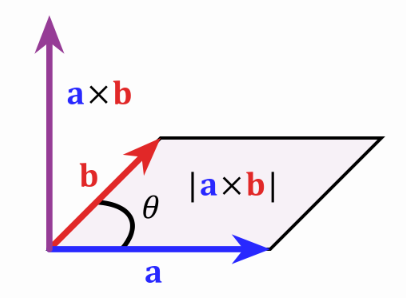​一般我们在解决立体几何题目时会选择建立坐标系，因为这样做比较保险也有固定套路。很多时候这些题目要求你计算某一个面的法向量（normal vector），这在高中阶段也是有固定方法的，我们这里想要介绍的是一种更高级也更迅速的方法，也就是引入向量叉乘（cross product，“向量”同物理中的“矢量”概念，一直想不通为啥数学和物理用不一样的名字，英文都是vector）这一概念。
我们都学过向量的标量积，也就是所谓的点乘（dot product），两个向量做标量积后得到的是一个标量。我们这里定义一种新的向量运算，也就是向量积或者叫叉乘: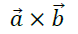其运算结果仍是一个向量，我们记之为向量c，它的模定义为：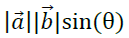其中θ为向量a和向量b的夹角，如下图所示，c的模即以a和b为两条边的平行四边形的面积。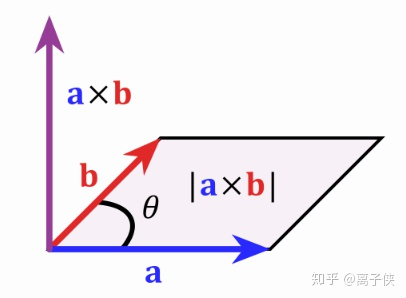图片来源：百度图片

c的方向定义为垂直于a和b所构成的平面，并且a，b和c构成右手螺旋定则，也就是右手四指方向从a转向b，大拇指即得到c方向。定义了这一新的运算之后，我们从这个定义出发能证明以下几条重要性质：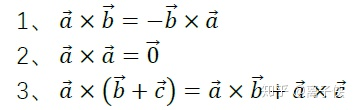前两条性质根据定义一眼就能看出，第三条叉乘的分配律是非常重要的性质，证明也比较困难，我们不打算赘述了。
那么在三维坐标系中，a和b拥有了坐标表示后，c的坐标该怎么计算呢？记x，y，z轴正方向的单位向量分别为i，j，k，则有：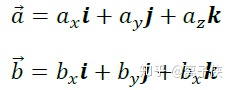则根据叉乘的上述三条性质我们得到c为：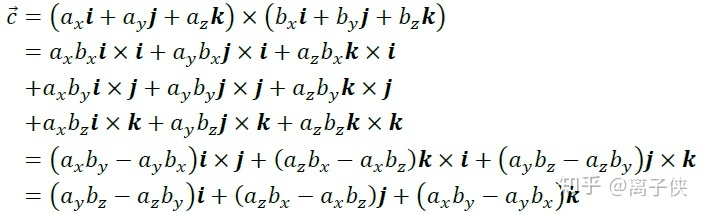第二个等号我们运用了性质3，第三个等号我们运用了性质1和性质2，最后一个等号则运用了简单的i，j，k之间的叉乘关系。这一计算公式让我们直接能够从a和b的坐标表示得到c的坐标表示，用行列式可以更简洁地表示并方便记忆：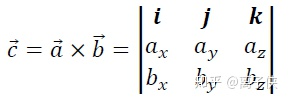有了这个公式，对于任意一个面的法向量，我们总可以选取该面上的两个不共线向量来直接叉乘出来，一般解题中直接就选该面的两条边上的单位向量就行了。
警告：这个方法比高中阶段介绍的方程求解法要方便许多，但是用这种方法解题万一出错了很可能得不到求解法向量这一步的过程分。

展开全文• 向量叉乘 a=(x1,y1)b=(x2,y2)a=(x_1,y_1)\quad b=(x_2,y_2)a=(x1​,y1​)b=(x2​,y2​) 点乘：a→⋅b→\overrightarrow{a}·\overrightarrow{b}a⋅b 得到实数，属于向量之间的标量运算 叉乘：a→×b→\...
向量叉乘

a

=

(

x

1

,

y

1

)

b

=

(

x

2

,

y

2

)

点乘：

a

→

⋅

b

→

\overrightarrow{a}·\overrightarrow{b}

得到实数，属于向量之间的标量运算叉乘：

a

→

×

b

→

\overrightarrow{a}\times\overrightarrow{b}

二维叉乘的数值概念为以两个向量为边所围成的平行四边形面积 集合概念为

a

→

与

b

→

\overrightarrow{a}与\overrightarrow{b}

的相对位置，右手定则得到补充知识

向

量

叉

乘

：

a

→

×

b

→

=

x

1

⋅

y

2

−

x

2

⋅

y

1

两

向

量

共

线

：

a

→

×

b

→

=

0

向

量

叉

乘

不

满

足

交

换

律

：

a

→

×

b

→

=

−

b

→

×

a

→

\begin{aligned} 向量叉乘：&\overrightarrow{a}\times\overrightarrow{b}=x_1·y_2-x_2·y_1\\ 两向量共线：&\overrightarrow{a}\times\overrightarrow{b}=0\\ 向量叉乘不满足交换律：&\overrightarrow{a}\times\overrightarrow{b}=-\overrightarrow{b}\times\overrightarrow{a} \end{aligned}

例子：：

(

a

−

b

)

→

×

(

a

+

b

)

→

=

2

×

(

a

→

×

b

→

)

证

明

：

(

a

−

b

)

→

×

(

a

+

b

)

→

=

a

→

×

a

→

+

a

→

×

b

→

−

b

→

×

a

→

−

b

→

×

b

→

=

2

×

(

a

→

×

b

→

)

几

何

意

义

:

平

行

四

边

形

对

角

线

为

边

的

平

行

四

边

形

面

积

为

原

平

行

四

边

形

面

积

的

两

倍

\begin{aligned} \overrightarrow{(a-b)}\times\overrightarrow{(a+b)}&=2\times(\overrightarrow{a}\times\overrightarrow{b})\\ 证明：\overrightarrow{(a-b)}\times\overrightarrow{(a+b)}&=\overrightarrow{a}\times\overrightarrow{a}+\overrightarrow{a}\times\overrightarrow{b}-\overrightarrow{b}\times\overrightarrow{a}-\overrightarrow{b}\times\overrightarrow{b}\\ &=2\times(\overrightarrow{a}\times\overrightarrow{b})\\ 几何意义&:平行四边形对角线为边的平行四边形面积为原平行四边形面积的两倍 \end{aligned}

三维空间叉乘表示为两向量围成的平面的法向量方向海伦公式证明
三角形面积公式

△

2

=

p

(

p

−

a

)

(

p

−

b

)

(

b

−

c

)

\triangle^2=p(p-a)(p-b)(b-c)证明：hdu多校第八场C题几何判断
Clockwise or Counterclockwise题目大意：
(0,0)为圆心，任意给出三点判断三点顺序为顺时针还是逆时针
可用二维叉乘意义表示 判断两两点相对位置
正数输出逆时针反之顺时针
#include<bits/stdc++.h>

#define ll long long
using namespace std;
ll t, x1, x2, x3, y1, y2, y3;
int main(){
scanf("%lld",&t);
while(t--){
scanf("%lld%lld%lld%lld%lld%lld", &x1, &y1, &x2, &y2, &x3, &y3);
if(x1 * y2 - x2 * y1 + x2 * y3 - x3 * y2 + x3 * y1 - x1 * y3>0)
printf("Counterclockwise\n");
else
printf("Clockwise\n");
}
return 0;
}

展开全文• 通过运算符重载，实现了二维向量的加减、点乘、叉乘运算。
•   先从简单的情况开始推导，考虑向量a⃗,b⃗,c⃗\vec{a},\vec{b},\vec{c}a,b,c在同一个平面，其中c⃗⊥a⃗\vec{c} \perp \vec{a}c⊥a，如下图所示，求取(a⃗×b⃗)×c⃗(\vec{a} \times \vec{b}) \times \vec{...线性代数 矩阵
• 文章目录3维向量的点乘叉乘运算三维向量的点乘三维向量叉乘点到直线的距离点到平面的距离 三维向量的点乘 点乘得到的是对应元素乘积的和，是一个标量，没有方向 V1( x1, y1, z1)·V2(x2, y2, z2) = x1x2 + y1y2 + ...线性代数
• 点乘的结果是是标量，点乘也被称为内积，是a向量在b向量上投影的长度与b向量的长度的乘积，反映了两个向量之间的相似度，两向量越相似，它们的点积就越大。 内积（点乘）的几何意义： 表征或计算两个向量之间的...向量点积
• 1.2D向量叉乘 2.3D向量叉乘 1.2D向量叉乘 U × V = Ux * Vy - Uy * Vx 2.3D向量叉乘 3D笛卡尔坐标系的个坐标轴两两垂直，并且有着如下的关系： x轴向量 × y轴向量=z轴向量 y轴向量 × z轴...
• ## 向量叉乘与叉乘矩阵

万次阅读 多人点赞 2017-02-22 19:04:41
本文以三维向量来说明向量的叉乘计算原理以及叉乘矩阵如何求取 1、向量叉乘的计算原理 a、b分别为三维向量： a叉乘b一般定义为：
• 在二维空间中，利用两个直线方程 y = kx + b 我们可以直接计算出交点，但是这种方法麻烦了些，并且套用到三维空间用公式就更麻烦了，接下来介绍的是如何利用向量叉乘求出直线交点。并且由于利用叉乘最后可以的到一个...
• 二维向量叉乘 A=(a1,a2) B=(b1,b2) A×B =(a1,a2)×(b1,b2) =a1b2-a2b1 三维向量叉乘 A=(a1,a2,a3) B=(b1,b2,b3) A×B =(a1,a2,a3)×(b1,b2,b3) =(a2b3-a3b2,a3b1-a1b3,a1b2-a2b1)线性代数
• 目录：质点：圆锥曲线题目的三维矢量解法在前两章我们指出，只有在矢量和对偶矢量之间才有自然的点乘。现在，我们要定义矢量和矢量间的点乘，为此我们必须引入一些附加结构。我们要引入的附加结构，是一个对称可逆的...
• [Unity游戏开发]向量在游戏开发中的应用（）博客中的案例源码。 本Demo使用的的开发工具是Unity5.0.1f1和VS2013，建议下载源码后用Unity5.0.1f1或者更高的版本打开。 本源码可以配合Sheh伟伟的博客来学习。
• ## 向量叉乘

万次阅读 多人点赞 2017-04-19 15:00:02
• 代数定义：设二空间内有两个向量a 和 b，定义它们的数量积（又叫内积、点积）为以下实数： 几何定义：设二空间内有两个向量a和 b ，它们的夹角为θ，则内积定义为以下实数： 通常我们在程序中使用点积来...
• 向量运算在游戏制作中经常用到，稍微总结一下。 一、点乘 如图，假设 向量a与b的点乘表示a在b上的投影与b的模的乘积 公式： function MathHelper....-- 向量叉乘 function MathHelper.GetV...lua 开发语言
• 　叉乘，也叫向量的外积、向量积。顾名思义，求下来的结果是一个向量，记这个向量为c。 |向量c|=|向量向量b|=|a||b|sin 向量c的方向与a,b所在的平面垂直，且方向要用“右手法则”判断（用右手的四指先表示向量a的...c
• 向量内积（点乘）和外积（叉乘）概念及几何意义 向量内积（点乘）和外积（叉乘）概念及几何意义
• 向量的叉乘即3D中任意两个三维向量叉乘（向量积）推导及代码实现
• 问题: 三维向量ℝ3R3\mathbb{R}^3上定义的叉积××\times是一种李括号，试证明
• 平面上个点:p1(x1,y1),p2(x2,y2),p3(x3,y3) s(p1,p2,p3)=(x1-x3)*(y2-y3)-(x2-x3)*(y1-y3) 如果s&gt;0 则说明 这连接这3个点时是按照逆时针的顺序, 如果是s&lt;0则说明连接这3个点是按照顺时针的顺序 ......